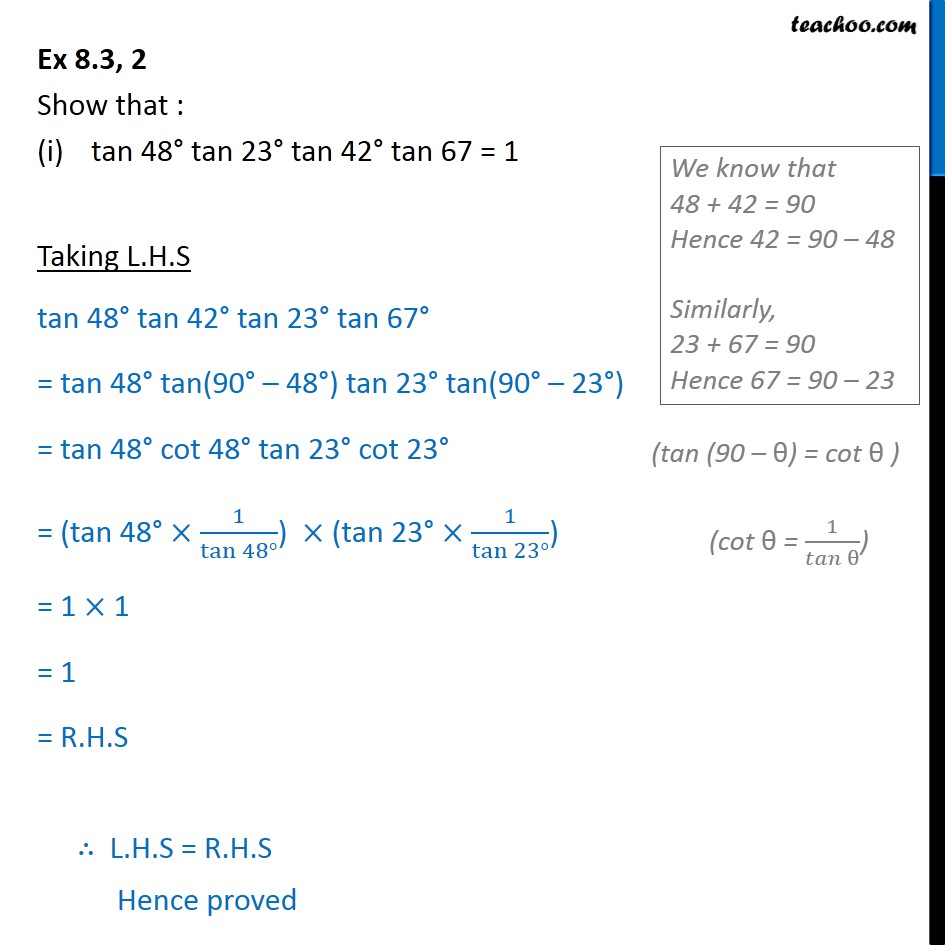1. Chapter 8 Class 10 Introduction to Trignometry (Term 1)
2. Concept wise
3. Trignometric ratios of complementry angles

Transcript

Ex 8.3, 2 Show that : tan 48 tan 23 tan 42 tan 67 = 1 Taking L.H.S tan 48 tan 42 tan 23 tan 67 = tan 48 tan(90 48 ) tan 23 tan(90 23 ) = tan 48 cot 48 tan 23 cot 23 = (tan 48 1/tan 48 ) (tan 23 1/tan 23 ) = 1 1 = 1 = R.H.S L.H.S = R.H.S Hence proved

Trignometric ratios of complementry angles